## Research Journal of Optics and Photonics

All submissions of the EM system will be redirected to Online Manuscript Submission System. Authors are requested to submit articles directly to Online Manuscript Submission System of respective journal.

Research Article, Res J Opt Photonics Vol: 2 Issue: 1

# Closed Form Wave Solution of Nonlinear Equations by Modified Simple Equation Method

Hossain AKMKS1*, Akbar MA2, Hossain MJ3 and Rahman MM4

1Department of Mathematics, Begum Rokeya University, Rangpur, Bangladesh

2Department of Applied Mathematics, University of Rajshahi, Rajshahi, Bangladesh

3Department of Mathematics, University of Rajshahi, Rajshahi, Bangladesh

*Corresponding Author : Hossain AKMKS
Department of Mathematics, Begum Rokeya University, Rangpur, Bangladesh
Tel: 1911764291
E-mail: kazi_bru@yahoo.com

Received: November 20, 2017 Accepted: January 04, 2018 Published: January 09, 2018

Citation: Hossain AKMKS, Akbar MA, Hossain MJ, Rahman MM (2018) Closed Form Wave Solution of Nonlinear Equations by Modified Simple Equation Method. Res J Opt Photonics 2:1.

## Abstract

The exploration of closed form wave solution of nonlinear evolution equation is being a interesting and more important subject area of research field in nonlinear physical sciences. Here we investigate the closed form wave solution of two important nonlinear equations named the third extended fifth order nonlinear equation and nonlinear telegraph equation. The obtained solutions of these two equations include an explicit function of the variables. It is revealed that the executed method is more affective for exploring the closed form wave solution of nonlinear evolution equations in mathematical physics. The method is remarkable of its very easy and short calculation procedure.

## Mathematics Subject Classifications (2010)

35C07, 35C08, 35C09, 35K05, 35P99

## Introduction

The study of the nonlinear evolution equations (NLEEs) and its closed form wave solutions has become very significant task in the recent years. The exploration of closed form wave solution is one of the easiest way to explains the evolution of the nonlinear wave phenomena that arises in the field of physical science and engineering. To better understand the inner mechanism of physical complex phenomena in nature, described by NLEEs, closed form wave solutions of NLEEs are essentially needed. In particular, the soliton solutions are very significant in the study of the models arising from various natural phenomena in scientific engineering fields such as optical fibers, high energy physics, nuclear physics, meteorology, geochemistry, elastic media, solid state physics, biophysics etc. Mathematical modeling of physical and engineering problems are inherently governed by nonlinear evolution equation (NLEEs). Therefore searching closed form wave solution of nonlinear evolution equations now becomes very important and interesting subject in nonlinear sciences. As a result, many authors who are concerned in nonlinear phenomena which exist in all fields of engineering and scientific works have been investigating the closed form wave solutions of nonlinear evolution equations. In recent times there are significant development have been made for exploring the closed form wave solutions of NLEEs and established many efficient and potential methods to solve the NLEEs for example the nonlinear transformation method , the sine-cosine method , the Exp-function method [3-6], the modified Exp-function , the Hirota’s bilinear transformation method [8,9], the variational method [10,11], the functional variable method , the modified simple equation method [13-18], the Adomian decomposition method , the extended direct algebraic method [20,21], the exp(- φ(η)) expansion method [22,23], the (G’/G) expansion method [24-30], the enhanced (G’/G) expansion method , the simplest equation method , the auxiliary ordinary differential equation method , the improve (G’/G) expansion method [34,35], the generalized Kudryashov method  etc. The recently developed modified simple equation method is getting attractiveness in use because of its straight forward and easy calculation procedure.

The aim of this paper to construct more closed form wave solutions of the nonlinear telegraph equation and the third extended fifth order nonlinear equation using the modified simple equation method. The remaining part of the paper is arranged as follows: In Section 2, method is described. In Section 3, the MSE method is applied to explore the solutions. In Section 4, graphical representation of the obtained solutions and In Section 5 conclusions are provided.

## Description of Modified Simple Equation (MSE) Method

To describe the modified simple equation method we consider a nonlinear equation of two variables of x and t in the following form:(1)

where P is polynomial of u(x, t) and its partial derivatives and u(x,t) is an unknown function.

The essential parts of this method are given in the following

Step 1: Introducing a compound variable ξ with the combination of real variables x and(2)

where c is the wave speed.

Equation (1) converts into an ODE with the help of Equation (2) of the following form:(3)

where Q is a polynomial in u(ξ) and its derivatives with respect to ξ.

Step 2: The solution of (3) can be expressed of the form:(4)

Where ψ(ξ) is not known and to be calculated such that ψ’(ξ)≠ 0 and αi(i=0,1,2,3,…N) are arbitrary constants to be determined such that αN ≠ 0. Since ψ(ξ) is not known function or not a solution of any equation so it is not possible to guess in advance what kind of solution may be obtained. This is the exceptionality of this method. Therefore it might be possible to achieve some fresh solution by this method.

Step 3: The positive integer N can be evaluated by balancing the highest order of derivative term and nonlinear terms appearing in (3).

Step 4: Calculate the derivatives u’,u”,...and place in Equation (4) into (3) then we find the function ψ(ξ). These make a polynomial of (1/ψ(ξ)) and now equating the like terms of this polynomial to zero gives a system of algebraic and differential equation which on solving we get the values of αi ’s and ψ(ξ)'

## Exploration of the Solutions

In this section, the modified simple equation method (MSE) has been put to use to examine the closed form solutions leading to solitary wave solutions to the third extended fifth order nonlinear equation and the nonlinear telegraph equation.

Example 1

Now we will apply the method which is described in section 2 to explore the closed form wave solution of nonlinear telegraph equation :(5)

Equation (5) is referred to as (1+1) dimensional hyperbolic telegraph equation with constant coefficients which models a mixture between diffusion and wave propagation by introducing a term that accounts for effects of finite velocity to standard heat or mass transport equation.

The wave transformation u(x,t)=u(ξ),ξ=x-ωt reduces the PDE (5) to ODE in the following form(6)

where ω is a constant (wave speed) to be determined latter.

Balancing between the highest order derivative term u’’and highest order nonlinear term u3 gives n = 1.

Hence the solution form of (6) turns into(7)

Where α0 and α1 are constant such that αi ≠ 0 and the unknown function ψ(ξ) to be calculated. Putting the value of (7) with its derivatives in equation (6) and equating the coefficient of ψ0, ψ-1, ψ-2, ψ-3 to zero we attain the following successive algebraic and differential equation,(8)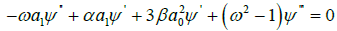(9)(10)(11)

By Equation 8 and (11) we achieve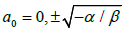and, since αi ≠ 0. From Equation 10 it can be figure out that(12)

Integrating Equation (12) with respect to ξ, yields(13)

and(14)

where c1 and c1 are arbitrary constants.

Case 1: When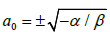and, solving equation (8) and (9) with (13) and (14), we achieveandthen substitute these values of α0, α1, ω and λ in (7) provides(15)

Hence in u(x,t) variables, the general closed form wave solution of nonlinear telegraph equation is as follows:(16)

As c1 and c2 are arbitrary constant one may randomly can choose their values. So if we let c1 = 1 and c2 = 1/λ then we get the following closed form wave solution(17)

Again setting c1 = -1 and c2 = 1/λ in Equation (16), we obtain the closed form wave solution of nonlinear telegraph equation of the form:(18)

Using hyperbolic functions identities, Equation (17) and Equation (18) can be rewritten as,(19)

and(20)

whereCase 2: When αi = 0 and, solving equation (18) and (19) with (13) and (14), we get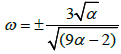andthen replace with these values of α0, α1, ω and λ in (17) provides the closed form wave solution in u(x,t) variables :(21)

Since c1 and c2 are arbitrary constant we may pick their values. If we replace c1 = 1 and c2 = 1/λ we get the closed form solution of nonlinear telegraph equation in the following form:(22)

Putting c1 = -1 and c2 = 1/λ in Equation (21) then the closed form wave solution is as follows

with hyperbolic functions characteristics, Equation (22) and Equation (23) can be modified as,(24)

and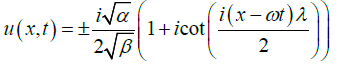(25)

Where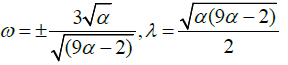Example 2

In this subsection we will use the modified simple equation method to search the closed form wave solution of the third extended fifth order nonlinear equation of the form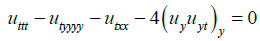(26)

The wave transformation u(x,t)=u(ξ),ξ = kx+ly-ωt transfers (26) to the ODE of the form(27)

Integrating (27) twice with respect to ξ and assuming the constant of integration to zero, we obtain(28)

Balancing between the highest-order derivative term u’’’ and the highest-order nonlinear term u’2 we get n=1.

Therefore, the solution of Equation (28) becomes(29)

Where ψ(ξ) is an unknown function to evaluated latter and α0 and α1 are constant such that αi ≠ 0. Substituting Equation 29 with its derivatives into (29) and then equating the coefficient of ψ0, ψ-1, ψ-2, ψ-3 to zero we get a system of successive algebraic and differential equation.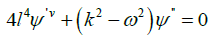(30)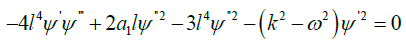(31)(32)(33)

From Equation (33), we obtain αi =3l.

And from Equation (30), we get(34)

Where c1, c2, c3 and c4 are arbitrary constants.

Solving Equation (31) by using (34) and αi =3l we achieve ω = ±k.

Case 1: When αi =3l and ω = k then c1 = c1+ c3(35)

Since c1 and c3 are constant so any one can put c1 = c1+ c3. The general closed form wave solution of third extended fifth order nonlinear equation is as follows:(36)

where ξ = kx+ly- ωt and ω = k.

Case 2: When αi =3l and ω = -k then(37)

Since c1 and c3 are integration constants, one may arbitrarily choose c1 = c1+c3.Thus, the general closed form traveling wave solution of the third extended fifth order non-linear equation is obtained as follows:(38)

where ξ = kx+ly- ωt and ω = -k

## Graphical Representation of the Obtained Solutions

In this section, we have explained the obtained solutions of the nonlinear telegraph equation and the third extended fifth order nonlinear equation. From the above we observed that the solution from Equation 15, 17 and 19 travelling wave solutions which are kink shape type wave solutions. The solutions shown in Equation 18, 20, 23 correspond to soliton solution. The solution (Equation 15) is represented in Figure 1. Which express the nature of kink shape wave solution with α=1, β=1, c1=1, c2=2 and the interval -5≤ x,t ≤ 5.The soliton solution (23) for α=1, β=1, c1=-1, and c2=1/λ within the interval -5≤ x,t ≤ 5. is given by Figure 2. The figures of others solutions are similar to kink type and soliton type and ignored these figures for simplicity. Again the solutions of third extended fifth-order nonlinear equation we also observed that the solutions (35) to (38) are traveling wave solutions which are all Singular kink wave solution. The solution (35) is represented in Figure 3. It shows nature of singular kink shape travelling solution with wave speed ω=5, a0=2, c1=1, c2=1,c3=1 and k=1 with the interval -5≤ x ≤ 5 and 0 ≤ t ≤ 4. The figures of solution 36 to 38 are similar to singular kink type and ignored these figures for simplicity (Figure 3).

Figure 1: Kink shape solution u(ξ) in (15) for α=1, β=1, c1=1, c2=2.

Figure 2: Soliton solution u(ξ) in (23) for α=1, β=1, c1=-1, and c2=1/λ.

Figure 3: Singular kink wave solution u(ξ) in (35) for ω=5, a0=2, c1=1, c2=1, c3=1 and k=1.

## Conclusion

In this article, the modified simple equation method has been successfully applied to explore the closed form wave solution of two nonlinear equation named the nonlinear telegraph equation and the third extended fifth order nonlinear equation. The solutions are verified to test the correctness by putting the solutions back into the original equation and found correct. The used method has many advantages: it is straightforward and calculation procedure is very concise. Therefore this efficient method could be more effectively used to solve various NLEEs which regularly arise in science, engineering and other technical arenas.

## Acknowledgements

The authors wish to take this chance to express their gratitude to the referees for their valuable observations and suggestions which improved the quality of this article.

## References